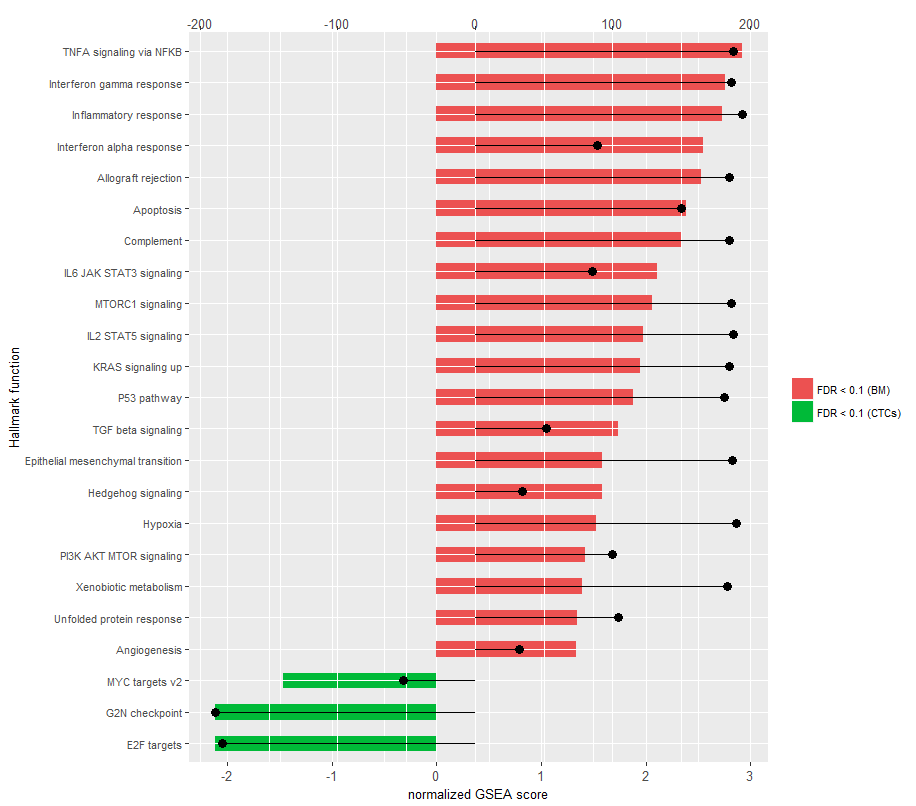Instantly share code, notes, and snippets.

Last active March 23, 2021 11:38
Star You must be signed in to star a gist
ggplot2 dual axes

## Dual axes for ggplot2

Modified from:

https://rpubs.com/kohske/dual_axis_in_ggplot2

Updated: 2016-07-27

### Example for x-axis

``````# Create fake data.frame
y1 = runif(100, 0, 100),
x1 = runif(100, 0, 100)
)

# Add second x-axis that scales with first

# Create plots
plot1.x = qplot(y = y1, x = x1, data = data.add.x)
plot2.x = qplot(y = y1, x = x2, data = data.add.x)

# Run function
ggplot_dual_axis(plot1.x, plot2.x, "x")
``````

### Example for y-axis

``````# Add second y-axis that scales with first

# Create plots
plot1.y = qplot(y = y1, x = x1, data = data.add.x)
plot2.y = qplot(y = y2, x = x1, data = data.add.x)

# Run function
ggplot_dual_axis(plot1.y, plot2.y, "y")
``````
This file contains bidirectional Unicode text that may be interpreted or compiled differently than what appears below. To review, open the file in an editor that reveals hidden Unicode characters. Learn more about bidirectional Unicode characters
 ggplot_dual_axis = function(plot1, plot2, which.axis = "x") { # Update plot with transparent panel plot2 = plot2 + theme(panel.background = element_rect(fill = NA)) grid.newpage() # Increase right margin if which.axis == "y" if(which.axis == "y") plot1 = plot1 + theme(plot.margin = unit(c(0.7, 1.5, 0.4, 0.4), "cm")) # Extract gtable g1 = ggplot_gtable(ggplot_build(plot1)) g2 = ggplot_gtable(ggplot_build(plot2)) # Overlap the panel of the second plot on that of the first pp = c(subset(g1\$layout, name == "panel", se = t:r)) g = gtable_add_grob(g1, g2\$grobs[[which(g2\$layout\$name=="panel")]], pp\$t, pp\$l, pp\$b, pp\$l) # Steal axis from second plot and modify axis.lab = ifelse(which.axis == "x", "axis-b", "axis-l") ia = which(g2\$layout\$name == axis.lab) ga = g2\$grobs[[ia]] ax = ga\$children[] # Switch position of ticks and labels if(which.axis == "x") ax\$heights = rev(ax\$heights) else ax\$widths = rev(ax\$widths) ax\$grobs = rev(ax\$grobs) if(which.axis == "x") ax\$grobs[]\$y = ax\$grobs[]\$y - unit(1, "npc") + unit(0.15, "cm") else ax\$grobs[]\$x = ax\$grobs[]\$x - unit(1, "npc") + unit(0.15, "cm") # Modify existing row to be tall enough for axis if(which.axis == "x") g\$heights[] = g\$heights[g2\$layout[ia,]\$t] # Add new row or column for axis label if(which.axis == "x") { g = gtable_add_grob(g, ax, 2, 4, 2, 4) g = gtable_add_rows(g, g2\$heights, 1) g = gtable_add_grob(g, g2\$grob[], 2, 4, 2, 4) } else { g = gtable_add_cols(g, g2\$widths[g2\$layout[ia, ]\$l], length(g\$widths) - 1) g = gtable_add_grob(g, ax, pp\$t, length(g\$widths) - 1, pp\$b) g = gtable_add_grob(g, g2\$grob[], pp\$t, length(g\$widths), pp\$b - 1) } # Draw it grid.draw(g) }

### ChongWu-Biostat commented Oct 12, 2016

It works pretty well. Thanks for writing this function!

### randomgambit commented Feb 2, 2017 • edited

hey thanks for this function but it does not seem to work very well. This is what I get running your code. As you can see there are some weird labels/texts on the upper right part of the chart. Any ideas what is causing this?### solo7773 commented Feb 20, 2017

helpful for me. thanks a lot

### ttnagata commented Mar 28, 2017

@randomgambit wrote:

hey thanks for this function but it does not seem to work very well. This is what I get running your code. As you can see there are some weird labels/texts on the upper right part of the chart

I had the same problem, for dual y-axis if you remove line 59 from the ggplot_dual_axis.R it works fine.

Also, for dual x-axis example apparently there are points plotted outside of the plot area, but if you remove line 51 it seems to work fine.

### FrancescoRan commented May 30, 2017

Thanks for the code! Do you have any suggestion on how perfectly overlap the panels of the two plots?

### ignacio82 commented Jul 25, 2017

Is it possible to add a label to the second x axis?

``````plot1.x = qplot(y = y1, x = x1, data = data.add.x)  + xlab('lab 1')
plot2.x = qplot(y = y1, x = x2, data = data.add.x) + xlab('lab 2')
ggplot_dual_axis(plot1.x, plot2.x, "x")
``````

Does not work

### jgarces02 commented Dec 7, 2017

Thanks a lot, @jslefche (and @ttnagata for corrections)! Very usefull!

### jgarces02 commented Dec 7, 2017

I agree with @FrancescoRan, is there any option to perfect overlap both plots? Because my zeros in every axis are in different position...### intelligentaccident commented Jun 29, 2018

It appears that changing line 59 to
`g = gtable_add_grob(g, g2\$grob[], pp\$t, length(g\$widths), pp\$b - 1)`
fixes the right-y-axis title issue
I also added this on line 2 to flip the text:
`plot2 <- plot2 + theme(axis.title.y = element_text(angle = 270)) `

### Sibojang9 commented Aug 31, 2018

Thanks to intelligentaccident. Your solution works perfectly.